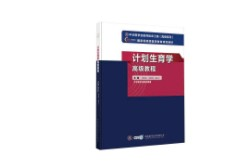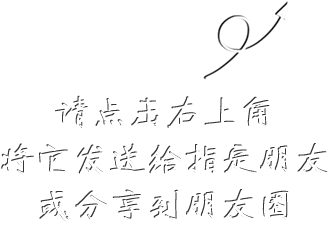999# 计划生育学高级教程

## ¥ 220.00220.00现货已售出0件

本书为《中华医学会医师培训工程（高级系列）》丛书之一，由中华医学会组织计划生育学权威专家编写。本书按照国家对高级卫生专业技术资格人员的专业素质要求，准确地介绍了计划生育学的基本理论和基本技术，重点阐述了计划生育学专业的基本理论和操作规范，力求反映目前计划生育学专业发展的国际规范和前沿动态，巩固和提高计划生育专科医师诊治、会诊、综合分析疑难病例及开展医疗先进技术的能力，同时还增加了测试题，作为考查医师对计划生育学专业知识掌握情况的依据。全书共 20 章，内容涵盖节育、生育及不育，附录还收录了常用宫内节育器、计划生育常用药物和检查、计划生育避孕节育相关网站及专业杂志等内容。本书实用性、指导性强，既可作为计划生育专科医师规范化培养的重要参考用书，也可作为拟晋升高级职称应试者的复习指导用书。

本书配有习题卡，题型分为单选题、多选题、共用题干单选题和病例分析题，可帮助读者深入理解相关理论，检测专业知识掌握程度，提高临床诊疗思维能力。试题全部由本书撰写专家亲自拟定。

• 删除
• 删除
• 删除
• 删除
• 删除
• 删除
• 删除
• 删除
• 删除
• 删除
• 删除
• 删除
• 删除
• 删除
• 删除
• 删除
• 删除
• 删除
• 删除
• 删除
• 删除
• 删除
• 删除
• 删除
• 删除
• 删除
• 删除
• 删除
• 删除
• 删除
• 删除
• 删除
• 删除
• 删除
• 删除
• 删除
• 删除
• 删除
• 删除
• 删除
• 删除
• 删除
• 删除
• 删除
• 删除
• 删除
• 删除
• 删除
• 删除
• 删除
• 删除
• 删除
• 删除
• 删除
• 删除
• 删除
• 删除
• 删除
• 删除
• 删除
• 删除
• 删除
• 删除
• 删除
• 删除
• 删除
• 删除
• 删除
• 删除
• 删除
• 删除
• 删除
• 删除
• 删除
• 删除
• 删除
• 删除
• 删除
• 删除
• 删除
• 删除
• 删除
• 删除
• 删除
• 删除
• 删除
• 删除
• 删除
• 删除
• 删除
• 删除
• 删除
• 删除
• 删除
• 删除
• 删除
• 删除
• 删除
• 删除
• 删除
• 删除
• 删除
• 删除
• 删除
• 删除
• 删除
• 删除
• 删除
• 删除
• 删除
• 删除
• 删除
• 删除
• 删除
• 删除
• 删除
• 删除
• 删除
• 删除
• 删除
• 删除
• 删除
• 删除
• 删除
• 删除
• 删除
• 删除
• 删除
• 删除
• 删除
• 删除
• 删除
• 删除
• 删除
• 删除
• 删除
• 删除
• 删除
• 删除
• 删除
• 删除
• 删除
• 删除
• 删除
• 删除
• 删除
• 删除
• 删除
• 删除
• 删除
• 删除
• 删除
• 删除
• 删除
• 删除
• 删除
• 删除